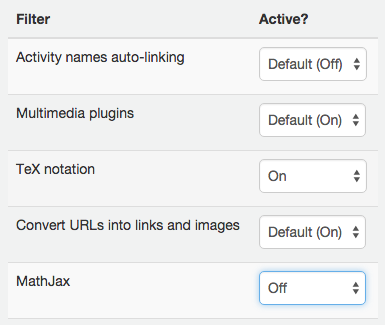## Writing in MathJax

MathJax will render LaTeX, MathML, and AsciiMath notation that works in all modern browsers enclosed in these delimiters:

• Inline formulas:  $$…$$
• Center aligned formulas:  $$…$$ or $…$

The complete MathJax documentation from mathjax.org

### Enabling the TeX notation filter

Our instance of Moodle uses the MathJax filter by default, but the TeX notation filter can be enabled at the course-level by doing the following:

1. Navigate to the course for which you want to enable the TeX notation filter.
2. Select the “More” tab, then click Filters.
3. Set “TeX notation” to “On.”
4. Set “MathJax” to “Off” to disable MathJax.
5. Click the [Save changes] button.Tagged in: